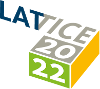#The 39th International Symposium on Lattice Field Theory (Lattice 2022)

Aug 8 – 13, 2022
Hörsaalzentrum Poppelsdorf
Europe/Berlin timezone

## Log-enhanced discretization errors in integrated correlation functions

Aug 11, 2022, 11:30 AM
20m
CP1-HSZ/0.002 (CP1-HSZ) - HS4 (CP1-HSZ)

### CP1-HSZ/0.002 (CP1-HSZ) - HS4

#### CP1-HSZ

50
Show room on map
Oral Presentation Standard Model Parameters

### Speaker

Rainer Sommer (DESY)

### Description

Integrated time-slice correlation functions $C(t)$ with weights $K(t)$ appear e.g. in
the moments method to determine $\alpha_s$ from heavy quark correlators,
in the muon g-2 determination or in the determination of smoothed spectral
functions. We show that the short distance part of the integral may lead to $\log(a)$-enhanced
discretization errors when $C(t)K(t) \sim t$ for small $t$. Starting from the Symanzik expansion
of the integrand we derive the asymptotic convergence of the integral at small lattice spacing.

For the (tree-level-) normalized moment $R_4$ of the heavy-heavy pseudo-scalar correlator $R_4$
we have non-perturbative results down to $a=10^{-2}$ fm and for masses, $m$, of the order of the charm
mass. A bending of the curve as a function of $a^2 m^2$ is observed at small lattice
spacings. We try to understand the behavior and extract an improved continuum limit.

### Primary authors

Leonardo Chimirri (HU Berlin & Desy Zeuthen) Rainer Sommer (DESY) Nikolai Husung (University of Southampton)